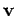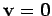Next: Newton's second law of Up: Newton's laws of motion Previous: Introduction

## Newton's first law of motion

Newton's first law was actually discovered by Galileo and perfected by Descartes (who added the crucial proviso in a straight line''). This law states that if the motion of a given body is not disturbed by external influences then that body moves with constant velocity. In other words, the displacementof the body as a function of timecan be written(91)

whereandare constant vectors. As illustrated in Fig. 14, the body's trajectory is a straight-line which passes through pointat timeand runs parallel to. In the special case in whichthe body simply remains at rest.

Nowadays, Newton's first law strikes us as almost a statement of the obvious. However, in Galileo's time this was far from being the case. From the time of the ancient Greeks, philosophers--observing that objects set into motion on the Earth's surface eventually come to rest--had concluded that the natural state of motion of objects was that they should remain at rest. Hence, they reasoned, any object which moves does so under the influence of an external influence, or force, exerted on it by some other object. It took the genius of Galileo to realize that an object set into motion on the Earth's surface eventually comes to rest under the influence of frictional forces, and that if these forces could somehow be abstracted from the motion then it would continue forever.Next: Newton's second law of Up: Newton's laws of motion Previous: Introduction
Richard Fitzpatrick 2006-02-02